Ex 7.5

Chapter 7 Class 12 Integrals
Serial order wise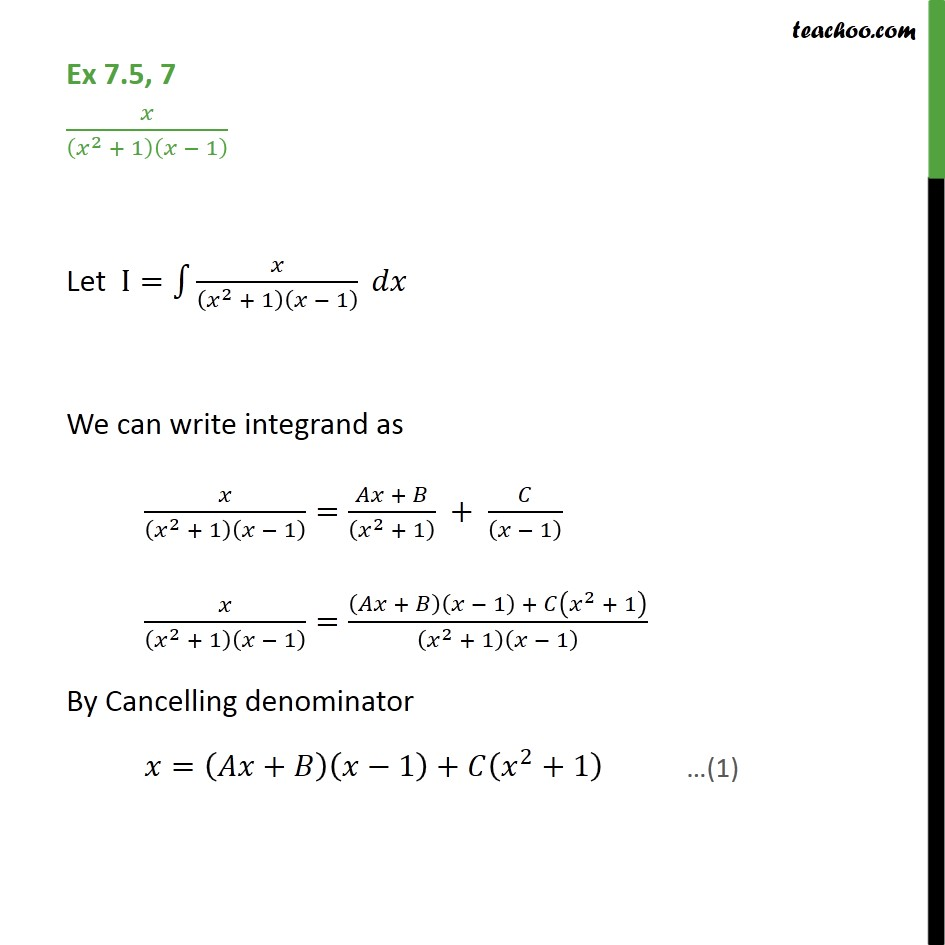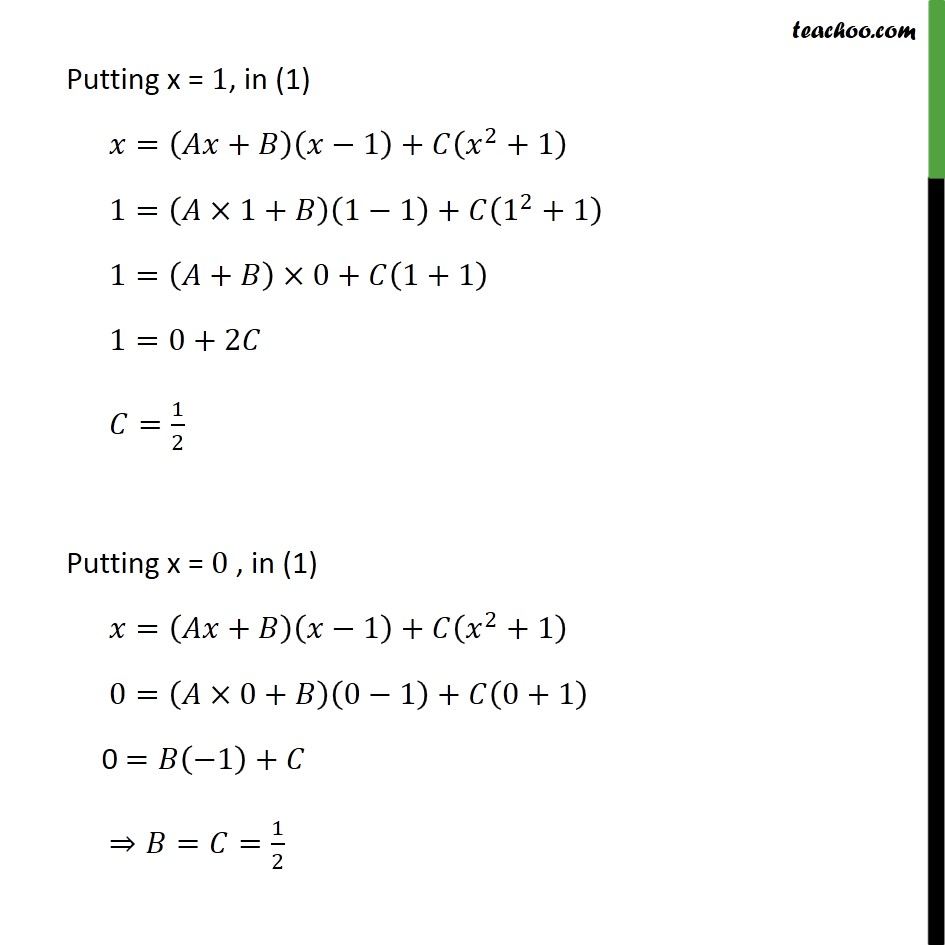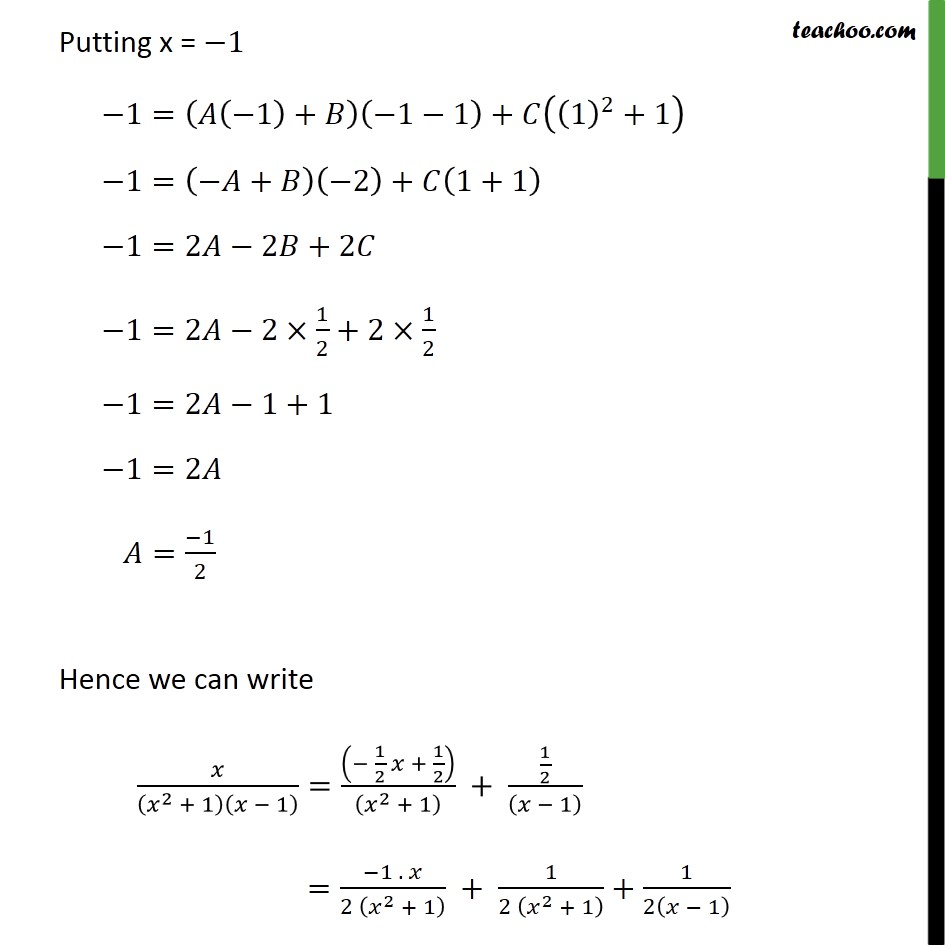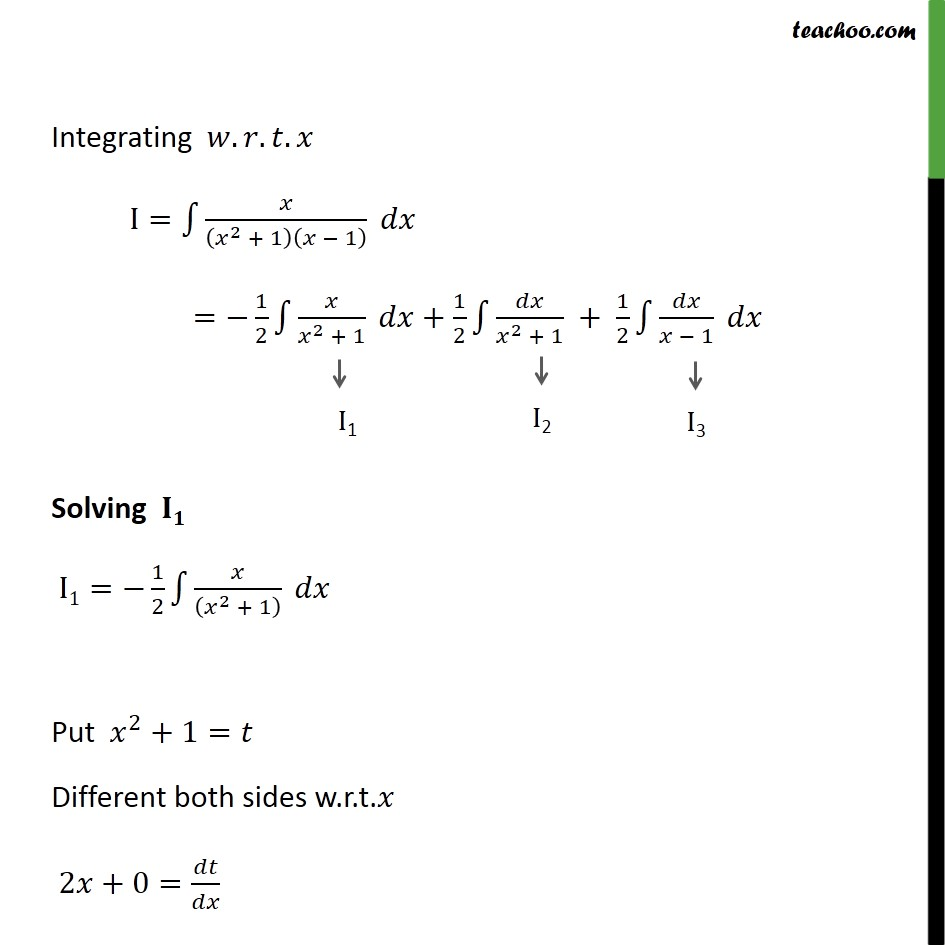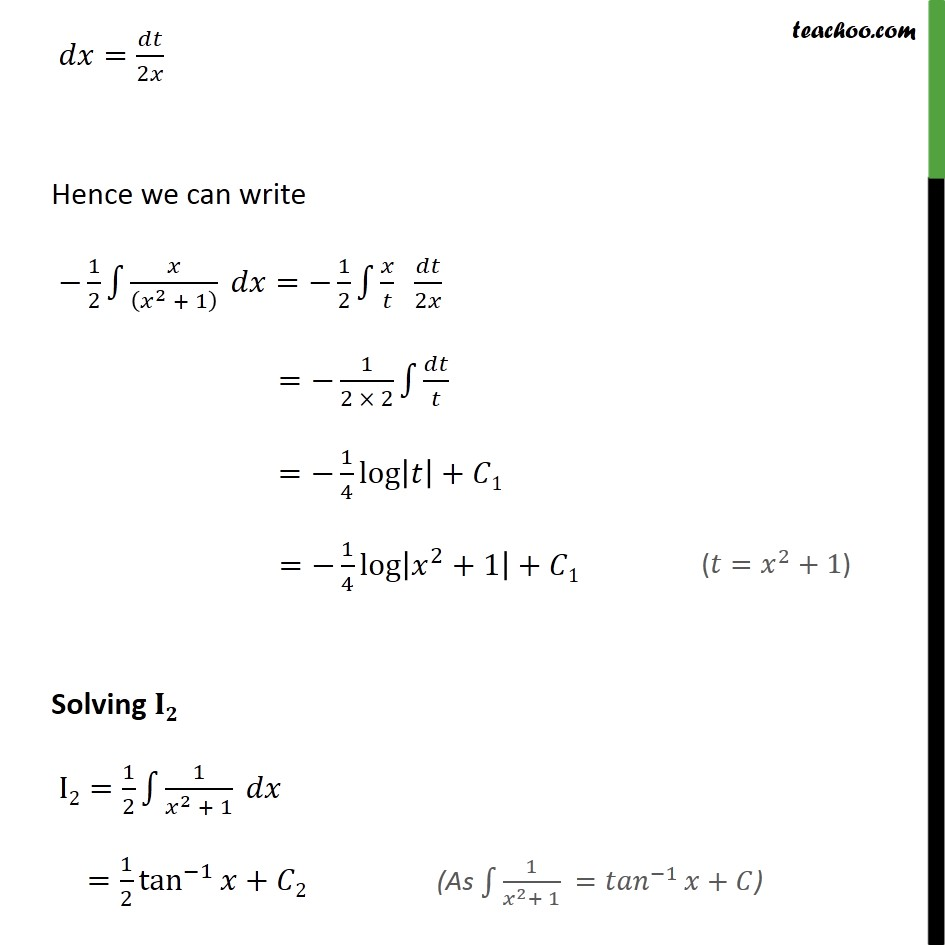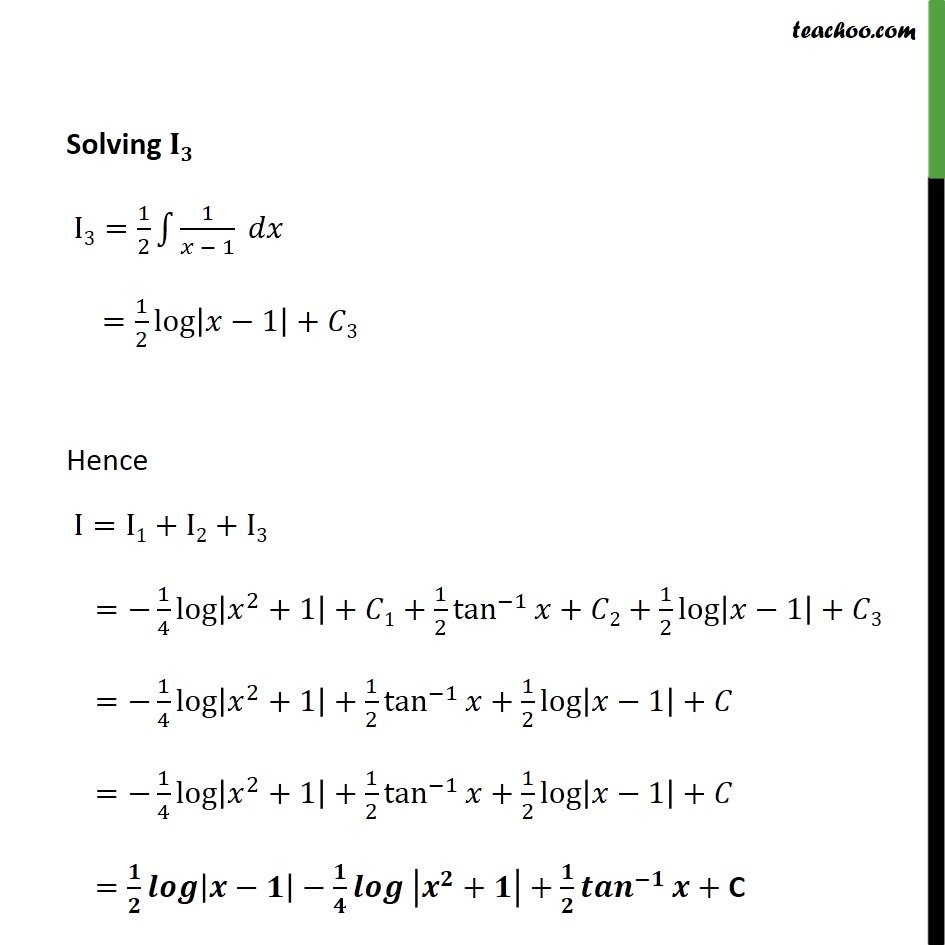Learn in your speed, with individual attention - Teachoo Maths 1-on-1 Class

### Transcript

Ex 7.5, 7 /( ^2 + 1)( 1) Let I= 1 /( ^2 + 1)( 1) We can write integrand as /( ^2 + 1)( 1) =( + )/(( ^2 + 1) ) + /(( 1) ) /( ^2 + 1)( 1) =(( + )( 1) + ( ^2 + 1))/( ^2 + 1)( 1) By Cancelling denominator =( + )( 1)+ ( ^2+1) Putting x = 1, in (1) =( + )( 1)+ ( ^2+1) 1=( 1+ )(1 1)+ (1^2+1) 1=( + ) 0+ (1+1) 1=0+2 =1/2 Putting x = 0 , in (1) =( + )( 1)+ ( ^2+1) 0=( 0+ )(0 1)+ (0+1) 0 = ( 1)+ = =1/2 Putting x = 1 1=( ( 1)+ )( 1 1)+ ((1)^2+1) 1=( + )( 2)+ (1+1) 1=2 2 +2 1=2 2 1/2+2 1/2 1=2 1+1 1=2 =( 1)/2 Hence we can write /( ^2 + 1)( 1) =(( 1/2 + 1/2))/(( ^2 + 1) ) + (1/2)/(( 1) ) =( 1 . )/(2 ( ^2 + 1) ) + 1/(2 ( ^2 + 1) )+1/2( 1) Integrating . . . I= 1 /( ^2 + 1)( 1) = 1/2 1 /( ^2 + 1) +1/2 1 /( ^2 + 1) + 1/2 1 /( 1) Solving I1= 1/2 1 /(( ^2 + 1) ) Put ^2+1= Different both sides w.r.t. 2 +0= / = /2 Hence we can write 1/2 1 /(( ^2 + 1) ) = 1/2 1 / /2 = 1/(2 2) 1 / = 1/4 log | |+ 1 = 1/4 log | ^2+1|+ 1 Solving I2=1/2 1 1/( ^2 + 1) =1/2 tan^( 1) + 2 Solving I3=1/2 1 1/( 1) =1/2 log | 1|+ 3 Hence I=I1+I2+I3 = 1/4 log | ^2+1|+ 1+1/2 tan^( 1) + 2+1/2 log | 1|+ 3 = 1/4 log | ^2+1|+1/2 tan^( 1) +1/2 log | 1|+ = 1/4 log | ^2+1|+1/2 tan^( 1) +1/2 log | 1|+ = / | | / | ^ + |+ / ^( ) + C